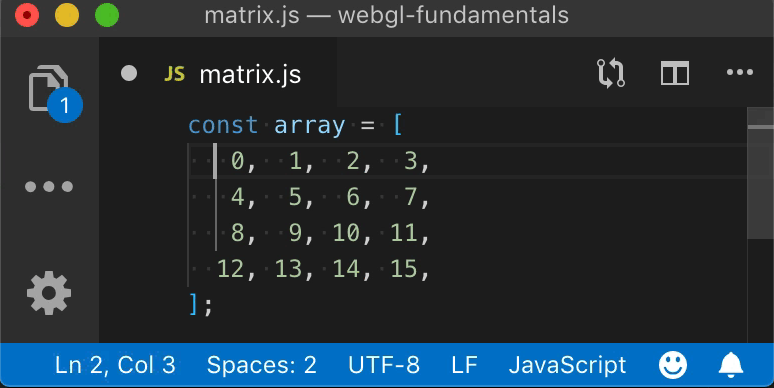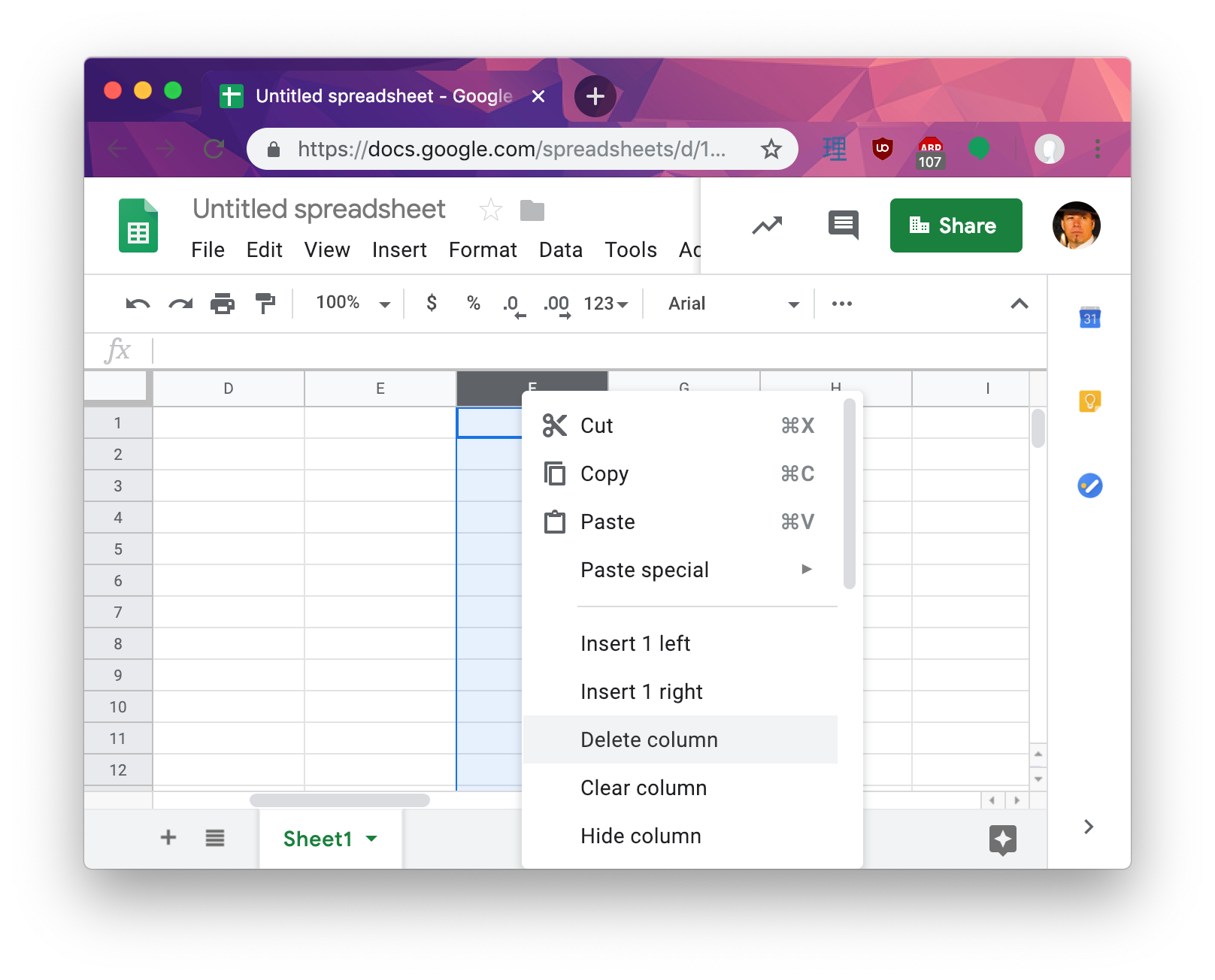# WebGLFundamentals.org

Fix, Fork, Contribute

# WebGL Matrices vs Math Matrices

This is article is an aside from the various articles that talk about matrices in particular the article that introduces matrices but also the article that introduces 3D, the article on perspective projection, and the article on cameras.

In programming generally a row goes from left to right, a column goes up and down.

## col·umn

/ˈkäləm/

noun

1. an upright pillar, typically cylindrical and made of stone or concrete, supporting an entablature, arch, or other structure or standing alone as a monument.

synonyms: pillar, post, pole, upright, vertical, ...

2. a vertical division of a page or text.

## row

/rō/

noun

• a horizontal line of entries in a table.

We can see examples in our software. For example my text editors shows Lines and columns, lines being another word for row in this case since column is already takenNotice in the bottom left area the status bar shows the line and column.

In spreadsheet software we see rows go acrossAnd columns go down.So, when we make a 3x3 or 4x4 matrix in JavaScript for WebGL we make them like this

``````const m3x3 = [
0, 1, 2,  // row 0
3, 4, 5,  // row 1
6, 7, 8,  // row 2
];

const m4x4 = [
0,  1,  2,  3,  // row 0
4,  5,  6,  7,  // row 1
8,  9, 10, 11,  // row 2
12, 13, 14, 15,  // row 3
];
``````

Clearly following the conventions above the first row of `m3x3` is `0, 1, 2` and the last row of `m4x4` is `12, 13, 14, 15`

As we see in the first article on matrices to make a fairly standard WebGL 3x3 2D translation matrix the translation values `tx` and `ty` go in locations 6 and 7

``````const some3x3TranslationMatrix = [
1,  0,  0,
0,  1,  0,
tx, ty,  1,
];
``````

or for a 4x4 matrix which is introduced in the first article on 3D the translation goes in locations 12, 13, 14 as in

``````const some4x4TranslationMatrix = [
1,  0,  0,  0,
0,  1,  0,  0,
0,  0,  1,  0,
tx, ty, tz,  1,
];
``````

But, there's an issue. Math conventions for matrix math generally do things in columns. A mathematician would write out a 3x3 translation matrix like thisand a 4x4 translation matrix like thisThat leaves us with a problem. If we want our matrices to look like math matrices we might decide to write a 4x4 matrix like this

``````const some4x4TranslationMatrix = [
1,  0,  0,  tx,
0,  1,  0,  ty,
0,  0,  1,  tx,
0,  0,  0,  1,
];
``````

Unfortunately doing it like that has issues. As mentioned in the article on cameras each of the columns of a 4x4 matrix often has a meaning

The first, second, and third columns are often considered the x, y, and z axis respectively and the last column is the position or translation.

One issue is in code it would be no fun to try to get those parts separately. Want the Z axis? You'd have to do this

``````const zAxis = [
some4x4Matrix,
some4x4Matrix,
some4x4Matrix,
];
``````

Ugh!

So, the way WebGL, and OpenGL ES on which WebGL is based, gets around this is it calls rows "columns".

``````const some4x4TranslationMatrix = [
1,  0,  0,  0,   // this is column 0
0,  1,  0,  0,   // this is column 1
0,  0,  1,  0,   // this is column 2
tx, ty, tz,  1,   // this is column 3
];
``````

Now it matches the math definition. Comparing to the example above, if we want the Z axis all we need to do is

``````const zAxis = some4x4Matrix.slice(8, 11);
``````

For those familiar with C++, OpenGL itself requires the 16 values of a 4x4 matrix to be consecutive in memory so in C++ we could create a `Vec4` struct or class

``````// C++
struct Vec4 {
float x;
float y;
float z;
float w;
};
``````

and we could create a matrix 4x4 from 4 of them

``````// C++
struct Mat4x4 {
Vec4 x_axis;
Vec4 y_axis;
Vec4 z_axis;
Vec4 translation;
}
``````

or just

``````// C++
struct Mat4x4 {
Vec4 column;
}
``````

And it would just appear to work.

Unfortunately it looks nothing like the math version when you actually declare one statically in code.

``````// C++
Mat4x4 someTranslationMatrix = {
{  1,  0,  0,  0, },
{  0,  1,  0,  0, },
{  0,  0,  1,  0, },
{ tx, ty, tz,  1, },
};
``````

Or back to JavaScript where we don't generally have something like C++ structs.

``````const someTranslationMatrix = [
1,  0,  0,  0,
0,  1,  0,  0,
0,  0,  1,  0,
tx, ty, tz,  1,
];
``````

So, with this convention of calling rows "columns" some things are simpler but others can be more confusing if you're a math person.

I bring all of this up because these articles are written from the POV of a programmer, not a math person. That means like every other single dimensional array that is treated as a 2 dimensional array, rows go across.

``````const someTranslationMatrix = [
1,  0,  0,  0,  // row 0
0,  1,  0,  0,  // row 1
0,  0,  1,  0,  // row 2
tx, ty, tz,  1,  // row 3
];
``````

just like

``````// happy face image
const dataFor7x8OneChannelImage = [
0, 255, 255, 255, 255, 255,   0,  // row 0
255,   0,   0,   0,   0,   0, 255,  // row 1
255,   0, 255,   0, 255,   0, 255,  // row 2
255,   0,   0,   0,   0,   0, 255,  // row 3
255,   0, 255,   0, 255,   0, 255,  // row 4
255,   0, 255, 255, 255,   0, 255,  // row 5
255,   0,   0,   0,   0,   0, 255,  // row 6
0, 255, 255, 255, 255, 255,   0,  // row 7
]
``````

and so these articles will refer to them as rows.

If you're a math person you might find it confusing. I'm sorry I don't have a solution. I could call what is clearly row 3 a column but that would also be confusing since it matches no other programming.

In any case, hopefully this helps clarify why none of the explanations look like something from a math book. Instead they look like code and use the conventions of code. I hope this helps explain what's going on and it's not too confusing for those who are used to math conventions.

• Fundamentals
• Image Processing
• 2D translation, rotation, scale, matrix math
• 3D
• Lighting
• Structure and Organization
• Geometry
• Textures
• Rendering To A Texture